# The Pentachoron

The pentachoron is the 4D equivalent of the tetrahedron. It consists of 5 regular tetrahedra joined at their faces, folded into 4D to form a 4D volume, meeting at 10 triangles, 10 edges, and 5 vertices. There are 3 tetrahedra surrounding every edge. It is also known as the 5-cell because it is made of 5 tetrahedral cells. Another name for it is the 4D simplex, so called because it is the simplest polychoron that encloses a non-zero 4D volume. It is the shape of Pento's pyramid in The Legend of the Pyramid.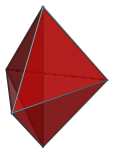## Cell-first projection

The cell-first perspective projection of the pentachoron into 3D is a tetrahedron, which is the nearest cell to the 4D viewpoint.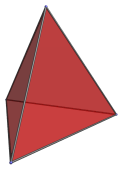The other 4 cells are on the far side of the pentachoron, and are not shown here.

## Vertex-first projection

The vertex-first projection of the pentachoron also has a tetrahedral envelope. This time, four of the cells are visible.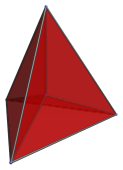The vertex at the center of this image, where the four internal edges meet, is actually the apex of the pentachoron pointing at us from the 4th direction. It is the nearest vertex to the 4D viewpoint.

The following images show the layout of these four cells in the projected image: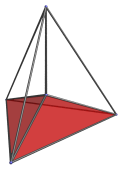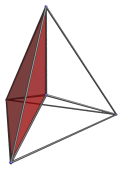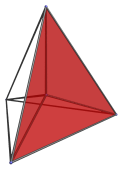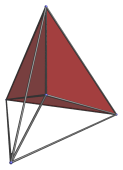The fifth cell is not visible here, as it lies on the far side of the pentachoron. It covers the entire tetrahedral volume of the projection.

Note that although the cells appear here as slightly flattened tetrahedra, this is only because they lie at an angle to the 4D viewpoint. In actuality, they are perfectly regular tetrahedra.

## Face-first projection

The next image shows the pentachoron viewed at face-first.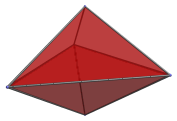This projection has a trigonal bipyramid as its envelope. There are two cells visible here, forming the upper and lower halves of the bipyramid, respectively. The following images show each of these two cells.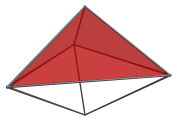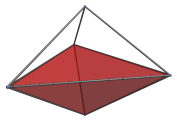The other 3 cells are not visible from this viewpoint, because they lie on the far side of the pentachoron.

These cells appear somewhat deformed from a regular tetrahedron, because they are all seen at an angle.

## Edge-first projection

The edge-first projection of the pentachoron also has a trigonal bipyramidal envelope.The vertical edge in this image is the closest edge to the 4D viewpoint. Three tetrahedral cells meet at this edge, as shown in the following images.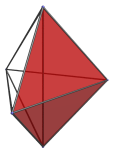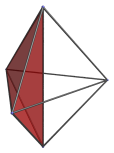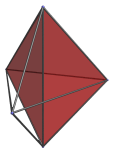## Coordinates

The coordinates of an origin-centered 5-cell with edge length 2 are:

• (1/√10, 1/√6, 1/√3, ±1)
• (1/√10, 1/√6, −2/√3, 0)
• (1/√10, −√(3/2), 0, 0)
• (−2√(2/5), 0, 0, 0)

Simpler coordinates can be obtained in 5D as all permutations of coordinates of:

• (0, √2, √2, √2, √2)

The 4D coordinates are derived by projecting these 5D coordinates back into 4D using a symmetric projection.

Last updated 17 Jun 2019.